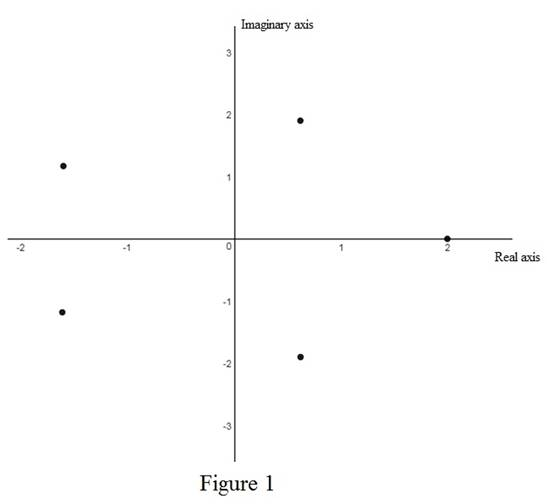# The roots of the fifths root of 32 and sketch the roots in the complex plane.### Single Variable Calculus: Concepts...

4th Edition
James Stewart
Publisher: Cengage Learning
ISBN: 9781337687805### Single Variable Calculus: Concepts...

4th Edition
James Stewart
Publisher: Cengage Learning
ISBN: 9781337687805

#### Solutions

Chapter I, Problem 38E
To determine

## To find: The roots of the fifths root of 32 and sketch the roots in the complex plane.

Expert Solution

The roots of fifth root of 32 are wk=118[cos(0+2kπ8)+isin(0+2kπ8)]=coskπ8+isinkπ8  where k=0,1,2,,7.

### Explanation of Solution

Theorem used:

Roots of a complex number:

Let z=r(cosθ+isinθ) and n be a positive integer. Then z has the n distinct nth roots wk=r1n[cos(θ+2kπn)+isin(θ+2kπn)] where k=0,1,2,,n1.

Calculation:

Rewrite the complex number 32 in polar form.

The polar form of the complex number z=a+bi is z=r(cosθ+isinθ) where r=|z|=a2+b2  and tanθ=ba.

Consider the complex number 32.

Obtain the argument of the complex number 32.

tanθ=032=0

Thus, the argument of the complex number 32 is θ=tan1(0)=0

Obtain the modulus of the complex number 32.

r=|32|=322+(0)2=322=32

Thus, the value of r=32.

Therefore, the polar form of the complex number 32 is 32=32(cos0+isin0).

By the above theorem, the roots of eighth root of 32 are wk=118[cos(0+2kπ8)+isin(0+2kπ8)]=coskπ8+isinkπ8 where k=0,1,2,,5.

Use online calculator to sketch the roots in the complex plane as shown below in Figure 1.From figure 1, it is observed that all fifths roots of 32 form a polygon on complex plane.

### Have a homework question?

Subscribe to bartleby learn! Ask subject matter experts 30 homework questions each month. Plus, you’ll have access to millions of step-by-step textbook answers!